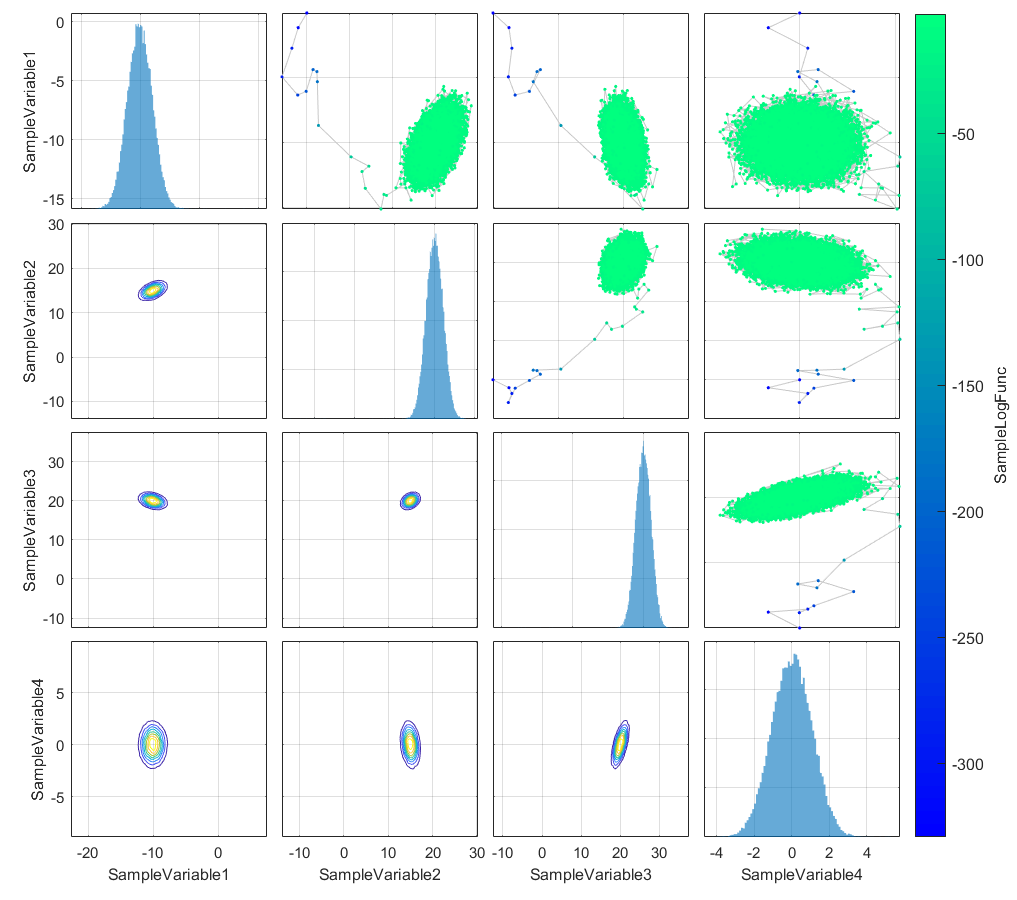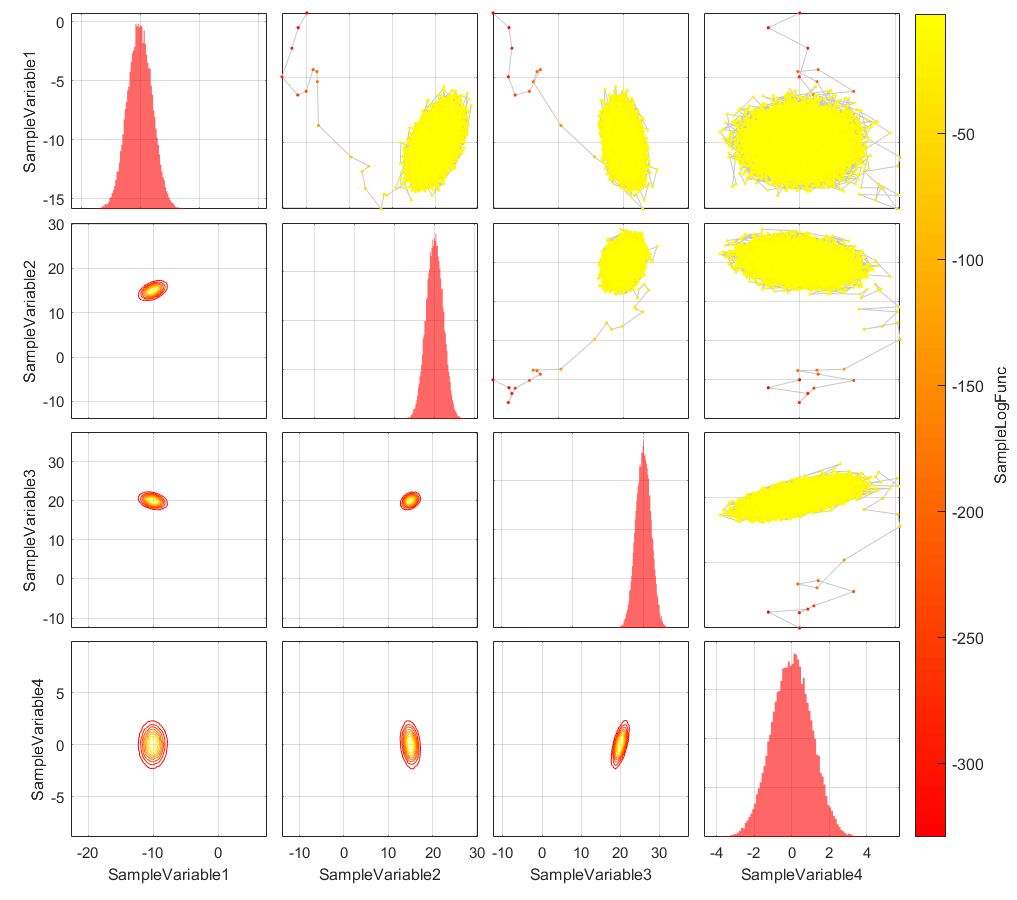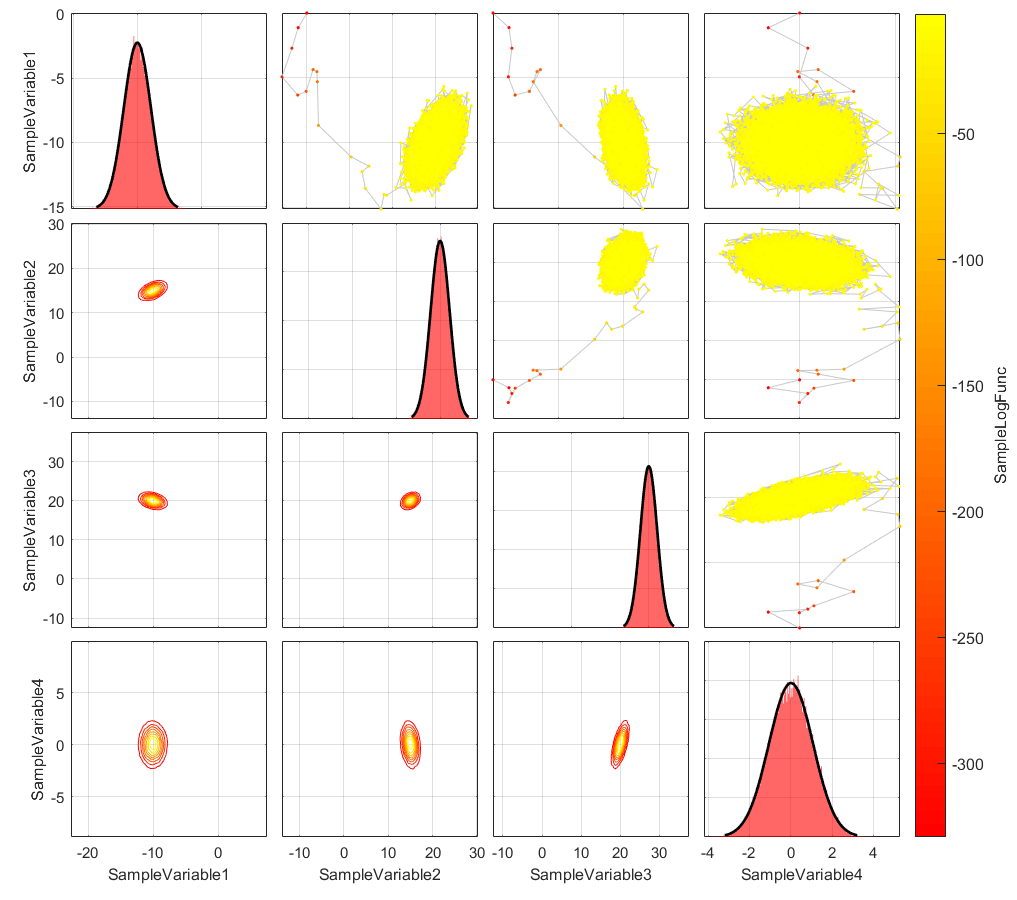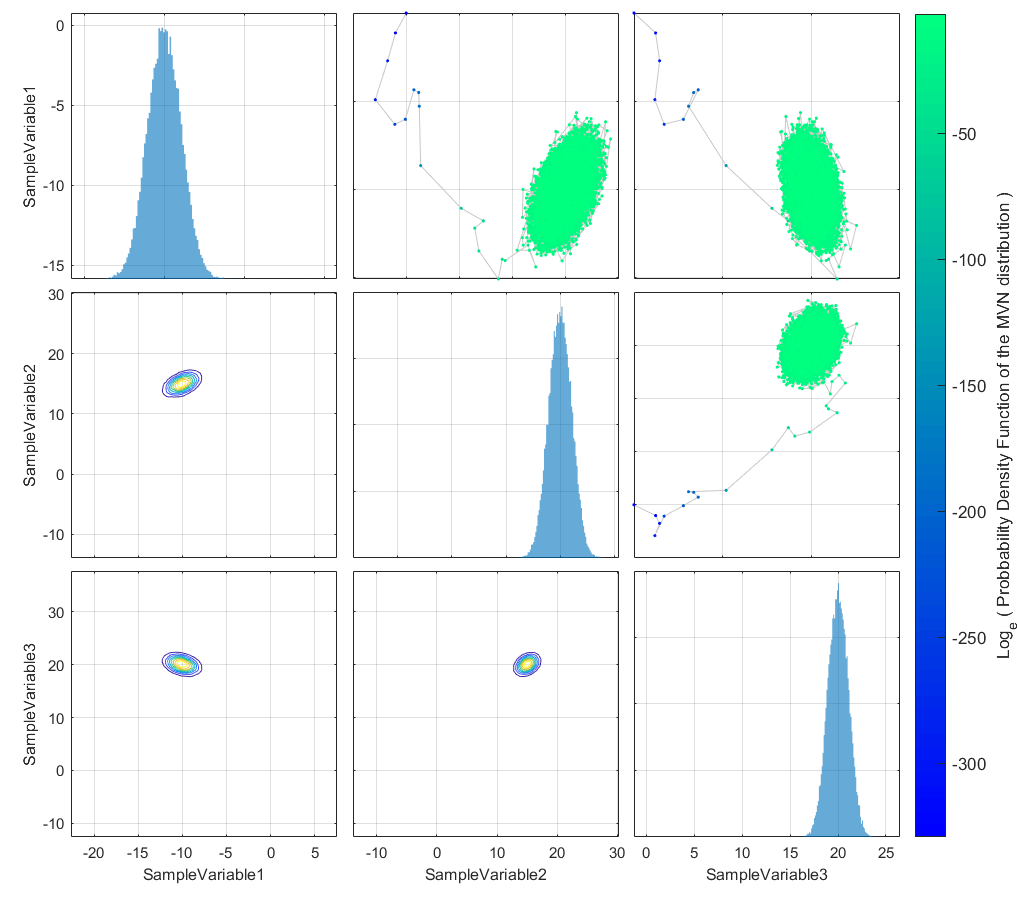Copyright (C) 2012-present, The Computational Data Science Lab
https://github.com/cdslaborg/paramonte

# Making a 2D grid of subplots with the ParaMonte visualization tools

NOTE
If you are viewing an HTML version of this MATLAB live script on the web, you can download the corresponding MATLAB live script visualization_grid.mlx file to this HTML page at,
https://github.com/cdslaborg/paramontex/tree/main/MATLAB/mlx
Once you download the file, open it in MATLAB to view and interact with its contents, which is the same as what you see on this page.
First, let's clean up the MATLAB environment and make sure the path to the ParaMonte library is in MATLAB's path list.
clc;
clear all;
close all;
format compact; format long;
%%%%%%%%%%%% IMPORTANT %%%%%%%%%%%%%
% Set the path to the ParaMonte library:
% Change the following path to the ParaMonte library root directory,
% otherwise, make sure the path to the ParaMonte library is already added
% to MATLAB's path list.
pmlibRootDir = './';
%%%%%%%%%%%%%%%%%%%%%%%%%%%%%%%%%%%%
% change MATLAB's working directory to the folder containing this script
% if MATLAB Live Scripts did not create a temporary folder, we would not
% have all of these problems!
try
setwdFilePath = websave("setwd.m","https://github.com/cdslaborg/paramontex/raw/main/MATLAB/mlx/setwd.m");
run(setwdFilePath); % This is a MATLAB script that you can download from the same GitHub location given in the above.
catch % alas, we will have to run the simulations in MATLAB Live Script's temporary folder
filePath = mfilename('fullpath');
[currentDir,fileName,fileExt] = fileparts(filePath); cd(currentDir);
cd(fileparts(mfilename('fullpath'))); % Change working directory to source code directory.
end

## ParaMonte's default visualization tools

The ParaMonte library ships with several visualization tools that automate much of the MATLAB coding required to visualize the output of the simulations performed by the ParMonte library samplers.
By replacing the input dataFrame to these tools and following the conventions of the ParaMonte library, one can also use these visualization tools for any dataset that may not have been generated by the ParaMonte library.
Consider the following Markov chain on the web in compact format generated by the ParaDRAM sampler of the ParaMonte library to sample a MultiVariate Normal distribution. Since this is a chain file as inidicated by its suffix "_chain.txt" We will read this file via the ParaDRAM sampler's readChain() method.
pm = paramonte();
This method automatically generates a set of tools that can be used to visualize the contents of the compact chain file. Note that these visualization tools are not unique to this particular method of the ParaDRAM sampler or other ParaMonte samplers. For the sake of illustration however, we will create plots using the above dataset read via readChain() method of the ParaDRAM routine.
chain = pmpd.chainList{1};
chain.plot.grid.make("columns", 8:11); % choose these column indices to draw from the dataFramegenerating subplot #1: (1,1) out of 16 generating subplot #2: (1,2) out of 16 generating subplot #3: (1,3) out of 16 generating subplot #4: (1,4) out of 16 generating subplot #5: (2,1) out of 16 generating subplot #6: (2,2) out of 16 generating subplot #7: (2,3) out of 16 generating subplot #8: (2,4) out of 16 generating subplot #9: (3,1) out of 16 generating subplot #10: (3,2) out of 16 generating subplot #11: (3,3) out of 16 generating subplot #12: (3,4) out of 16 generating subplot #13: (4,1) out of 16 generating subplot #14: (4,2) out of 16 generating subplot #15: (4,3) out of 16 generating subplot #16: (4,4) out of 16
Be default, the visualization tools are loaded with a set of predefined settings. For example, ParaMonte visualizations are by default colored (unless mutiple variables are to be displayed). These however, can be readily changed. For example, to change the colormap,
chain.plot.grid.colormap
ans = struct with fields:
enabled: 1 values: []
chain.plot.grid.colormap.values = autumn;
chain.plot.grid.template.histogram.histogram.kws.faceColor = "red";
chain.plot.grid.make()generating subplot #1: (1,1) out of 16 generating subplot #2: (1,2) out of 16 generating subplot #3: (1,3) out of 16 generating subplot #4: (1,4) out of 16 generating subplot #5: (2,1) out of 16 generating subplot #6: (2,2) out of 16 generating subplot #7: (2,3) out of 16 generating subplot #8: (2,4) out of 16 generating subplot #9: (3,1) out of 16 generating subplot #10: (3,2) out of 16 generating subplot #11: (3,3) out of 16 generating subplot #12: (3,4) out of 16 generating subplot #13: (4,1) out of 16 generating subplot #14: (4,2) out of 16 generating subplot #15: (4,3) out of 16 generating subplot #16: (4,4) out of 16
To draw the 2d grid of subplots kernel density estimate plots, the ParaMonte visualizer utilizes a wide array of MATLAB builtin plotting tools. The fundamental types of plots that can be added to the individual subplots in grdi plot and their properties are listed in the template component of the grid plot object. Set the properties of different types of subplots via this component.
The types of plots that are drawn in the upper and lower triangles of the grid plot as well as its diagonal subplots are given in the plotType component of the grid plot. For example,
chain.plot.grid.plotType
ans = struct with fields:
upper: [1×1 struct] lower: [1×1 struct] diag: [1×1 struct]
chain.plot.grid.plotType.diag
ans = struct with fields:
enabled: 1 value: "histogram" names: ["histogram" "histfit"]
chain.plot.grid.plotType.diag.names % these are the names of possible plot types that can appear in the diagonal elements of the grid plot.
ans = 1×2 string array "histogram" "histfit"
chain.plot.grid.plotType.diag.value % this is the name of the current plot type in the diagonal subplots.
ans = "histogram"
chain.plot.grid.plotType.diag.value = "histfit"; % choose histfit for the diagonal subplots
This will cause the diagonal elements of the grid plot to become histograms with distribution fits via MATLAB's builtin histfit() function. By default, the fitted distribution is "Normal", but this can be changed if needed,
chain.plot.grid.template.histfit.histfit.dist
ans = "Normal"
chain.plot.grid.colormap.values = "autumn";
chain.plot.grid.make();
generating subplot #1: (1,1) out of 16 generating subplot #2: (1,2) out of 16 generating subplot #3: (1,3) out of 16 generating subplot #4: (1,4) out of 16 generating subplot #5: (2,1) out of 16 generating subplot #6: (2,2) out of 16 generating subplot #7: (2,3) out of 16 generating subplot #8: (2,4) out of 16 generating subplot #9: (3,1) out of 16 generating subplot #10: (3,2) out of 16 generating subplot #11: (3,3) out of 16 generating subplot #12: (3,4) out of 16 generating subplot #13: (4,1) out of 16 generating subplot #14: (4,2) out of 16 generating subplot #15: (4,3) out of 16 generating subplot #16: (4,4) out of 16
for i = 1:length(chain.plot.grid.columns) % now change the colors of hisfit subplots to red.
chain.plot.grid.currentFig.subplotList{i,i}.currentFig.histfit{1}(1).FaceColor = "red";
endTo reset the properties of the plot object to the default settings, try,
chain.plot.grid.reset();
ParaDRAM - NOTE: resetting the properties of the grid plot...
To reset the entire plot object including reading the data again from the input dataFrame, try,
chain.plot.grid.reset("hard");
ParaDRAM - NOTE: creating the grid plot object from scratch...
Similarly, to change the properties of the colorbar, try,
chain.plot.grid.colorbar.kws
ans = struct with fields: fontSize: 12
chain.plot.grid.colorbar.kws.fontSize = 13;
Remember that a handle to all objects in the plot is also stored in the currentFig component of the object. Most of the properties of the figure, axes, and the plots can be also changed directly via these handles. For example, to set the label to somethign other than the default (which is taken from the ccolumn component's value), one could try,
chain.plot.grid.make("columns", 8:10);
generating subplot #1: (1,1) out of 9 generating subplot #2: (1,2) out of 9 generating subplot #3: (1,3) out of 9 generating subplot #4: (2,1) out of 9 generating subplot #5: (2,2) out of 9 generating subplot #6: (2,3) out of 9 generating subplot #7: (3,1) out of 9 generating subplot #8: (3,2) out of 9 generating subplot #9: (3,3) out of 9
chain.plot.grid.currentFig.colorbar.Label.Interpreter = "tex";
chain.plot.grid.currentFig.colorbar.Label.FontSize = 13;
chain.plot.grid.currentFig.colorbar.Label.String = "Log_e ( Probbability Density Function of the MVN distribution )";## Plotting only one traingle of the grid plot

It is possible to disable some part of the grid plot via the plotType attribute of the grid plot. For example, to disable the upper plot and show only lineScatter plots on the lower half,
chain.plot.grid.reset();
ParaDRAM - NOTE: resetting the properties of the grid plot...
chain.plot.grid.plotType.upper.enabled = false;
chain.plot.grid.plotType.lower.value = "lineScatter";
chain.plot.grid.make("columns", ["SampleVariable1",9:10]);